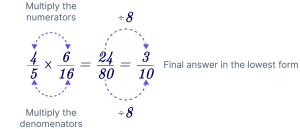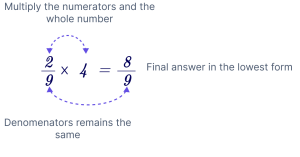# Multiplying Fractions Calculator

Multiplying Fractions Calculator by The Mathematics Master is a free online calculator that shows the result of multiplying two fractions. The online multiplying fractions calculator tool expedites the calculation, showing the result in a flash of time.

$$\dfrac{A}{B} \times \dfrac{C}{D} = ?$$

## What is Meant by Multiplying Fractions?

The ratio of two numbers in mathematics is defined using fractions. A fraction is, in other words, a numerical value that is not a whole number. The fraction can be written as a/b, where a and b are the numerator and denominator, respectively.

The fraction can be categorized as proper, improper, or mixed. If the two fractions are a/b and c/d, then the formula for multiplying two fractions is as follows:

$$(\dfrac{a}{b}) . (\dfrac{c}{d}) = \dfrac{ac}{bd}$$

Where: a, b, c, and d are integers.

Fractions are multiplied by multiplying the given numerators first and then by multiplying the specified denominators. The final step is to simplify the resultant fraction further and, if necessary, reduce it to its simplest form.

## Rules of Multiplying Fractions

The following rules should be followed while multiplying fractions:

1. If there are any mixed fractions, you must first convert them to improper fractions. And then, the numerators of the fractions should be multiplied.
2. Separately multiply the denominators.
3. Reduce the value to its simplest form.

Any two fractions can have their product determined by using these three rules.

## Example of Multiplying Fraction by Fraction

To multiply two fractions, either proper or improper, follow these steps:

1. Multiply the numerators
2. Multiply the denominators
3. Simplify the product## Example of Multiplying Fraction by Whole Number

To multiply a proper or improper fraction by a whole number, follow these steps:

1. Multiplying the numerator by the whole number
2. The denominator stays the same
3. Reduce the product to its simplest form.## FAQs

How to put a fraction in a calculator?

Even using a calculator can be challenging to calculate fractional values. A calculator’s fraction button can allow you to enter a fraction there. If your calculator does not have this capability, you may use an online fraction calculator.

How to Multiply Fractions with Mixed Numbers?

Simplify the fraction if it needs to be multiplied by a mixed number (fraction). Then, multiply the numerators and the denominators by the numerators once the mixed fraction has taken the shape of $$\dfrac{p}{q}$$.